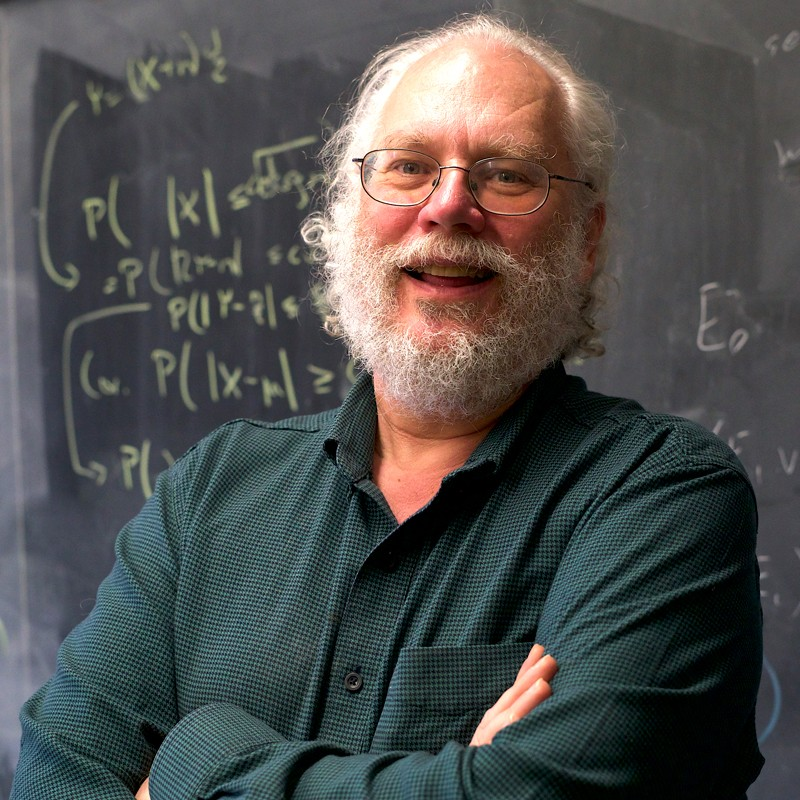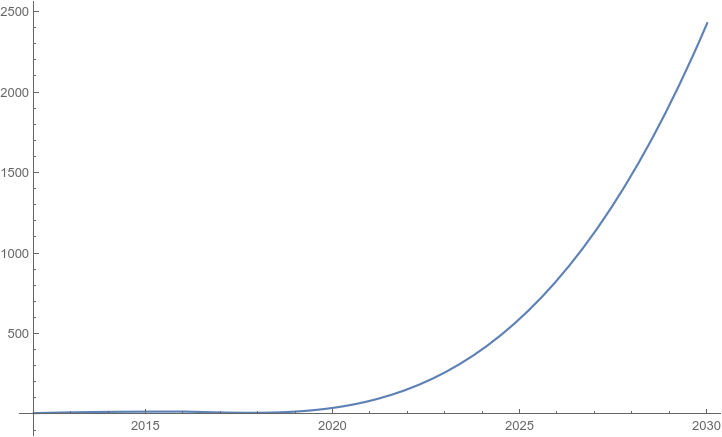Shor's algorithm

Summary

• This algorithm was created to solve problems of integer factorization and discrete logarithm computation in a finite group. Developed by Peter Shor in 1994, it allows factorizing N in polynomial time (O(log3N)) using O(log N) qubits;
• Technically, it means that it would take a quantum computer with around 3000 qubits just slightly longer to hack into an RSA cryptographic system with a 2048-bit key than the initial encryption time;
• Similarly, DSA, EdDSA and several other asymmetric cryptographic systems vulnerable to quantum attacks could be compromised. Note that Shor's algorithm does not affect symmetric ciphers (AES, etc.) and hash functions (SHA, etc.), since there are other less efficient algorithms used to analyze them.
More on the topic
The American mathematician Peter Shor developed a quantum algorithm for integer factorization, later named after him, in 1994. The algorithm uses the function period-finding method and can be adjusted to solve problems of discrete logarithm computation in a finite group or a group of elliptic curve points.

The efficiency of Shor's algorithm was proven in 2001 when a quantum computer with seven qubits factorized the integer 15.

If a quantum computer of sufficient power appears, Shor's algorithm will efficiently (i.e. only slightly slower than the required encryption time) compromise most asymmetric cryptographic schemes used nowadays, such as RSA, DSA, EdDSA, etc.).Peter Shor
The table below shows the current (as of early 2021) best results for the factorization of numbers used as public keys in the most common public-key cryptosystem, RSA. Best factorization results for classical computers are also presented for comparison.
The figure below shows the graph of the extrapolation function based on the second column of the table (X-axis represents the year, Y-axis – the number of bits in the RSA numbers factorized by the quantum computer). Considering that nowadays, data protection experts suggest using at least RSA-2048 (RSA-3072 is still more preferable), one can conclude that quantum computers capable of efficiently analyzing current cryptosystems will appear between 2028 – 2033.Fig.1. Extrapolation of quantum computer capabilities for RSA number factorization.
Note that some of the results shown in the table have been obtained by implementing models previously considered not quite suitable for cryptanalysis, such as the quantum annealing model implemented on D-Wave quantum computers.

Shor's algorithm does not affect symmetric ciphers (AES, etc.) and hash functions (SHA, etc.), since there are other less efficient algorithms (Grover's, Simon's, BHT etc.) used to analyze them. In this case, efficient protection means simply increasing parameters by 2-3 times.
However, since modern telecommunications networks almost always use symmetric algorithms alongside asymmetric ones (as in the TLS protocol), Shor's algorithm could pose a real threat to the entire cryptographic landscape. An adversary can already have traditionally encrypted data backed up, waiting to decrypt it once they get access to a quantum computer.

To counter the quantum threat, the global cryptographic community, including the QApp team, is working to develop and implement quantum-resistant cryptographic algorithms.